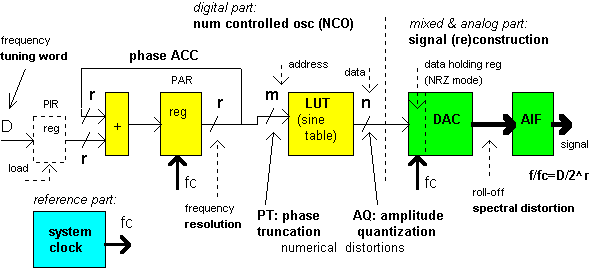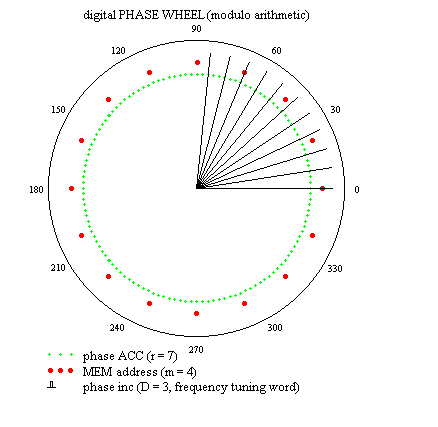DDS: background   |  numerical distortion   |  simulations (Mathcad)   |  products

DDS  technology

"The future of agile signal synthesis lies in DDS ."  [AnaDev]
"There is no longer one DDS aspirin for all system headaches."  [Sciteq]

Direct Digital Frequency Synthesis (DDFS or simply DDS), also known as Numerically Controlled Oscillator (NCO), is a technique using digital-data and mixed/analog-signal processing blocks as a means to generate real-life waveforms that are repetitive in nature. It is used especially for a precise, fast frequency and phase tunable output. DDS solutions can be implemented in LSI (large-scale integration) and they play an ever-increasing role in digital waveform and agile clock generation, and modulation.
As a discrete-time and discrete-amplitude system, DDS roots from sampling and quantizing theorems.

NCO-based DDS is a point(memory location)-skipping technique (and a constant interpolation of the stored signal) and runs at constant update(clock)-rate. As the DDS output frequency is increased, the number of samples per waveform cycles decreases.
This method is not to be confused with the common sample buffer playback, which is the traditional PPC (point-per-clock) synthesis at variable clock-rate.
Fig. 1 shows the basic block diagram of a generic DDS system design.Fig. 1 The standard DDS structure

The DDS architecture can be viewed as a simple assembly containing only three parts:

(1) fixed-rate system clock - the time reference part

(2) NCO - the digital part: generating numerical samples of (sine) waveform at clock rate

• ACC: phase ACCumulator ( register length: r - bit ), called also digital Frequency-to-Phase Converter (FPC), or digital phase wheel ( phase pointer: digital representation of instantaneous phase, see Fig. 2 ).

• For linear phase samples, overflowing ACC formed by an adder and PAR (phase accumulator register) integrates at-a-clock-rate the frequency tuning data in PIR (phase increment register). The frequency setting value added to ACC controls the average frequency of the "overflowing (wrapping) event".
Note: the ACC width ( r ) determines the frequency resolution of the DDS.

LUT: Look-Up Table ( memory address length: mdata length [memory width]: n ), called also digital Phase-to-Amplitude Converter (PAC), or polar-to-rectangular transformation (projection of the real or imaginary component in time), or (sine) waveform mapping device - a MEMory.
Here we model all techniques of calculating data as simple lookup table operations.
The lookup memory contains one cycle of the waveform to be generated.
For practical considerations, only the high bits of the ACC are sent to index the LUT ( the size of the LUT is 2m words,  phase truncation: m < r ). LUT translates truncated phase information, being in digital form ( see Fig. 2 ), into quantized numerical waveform samples - this is the most challenging part of NCO.
Numerical distortions (algorithmic nonlinearities) due to finite-wordlenght effects present a major contribution to system complexity.
Note: phase resolution (m - bit) has to match with the amplitude precision (n - bit).

NCO is actually a very simple toolbox and while it is most straightforward to be realized as hardware, it can also  be implemented in software. Effectively, NCO is a specialized calculator (in modulo arithmetic), optimized for repeated calculations of numeric samples of the wave.
The NCO structure supports phase-continuous digital modulation techniques.
DDS is a numeric - rather than a digital - technique and using this term helps to dissociate DDS from digital circuits’ unsavory reputation.Fig. 2 Each red-point (MEM address) on the phase wheel corresponds to
the equivalent point on a cycle of (sine) waveform.
Phase error introduced by approximation (truncated ACC) results in
periodic error in time (hence line spectra occurs in frequency) during the PAC process.
Movie Clip: pACC.avi (790 KB)  )

(3) (Re)construction of analog (sine)wave - the mixed-signal and analog part: recovering the signal from its numerical samples

• DAC: Digital-to-Analog Converter ( data length: n - bit ), called also number-to-voltage(current) converter, realises a hybrid multiplication (the DAC function).

• The amplitude number sequence (the output of NCO) is fed to DAC. Usually in NRZ (non-return-to-zero) mode, there is a roll-off effect of ZOH (zero-order-hold): sinx/x spectral envelope.
The actual realization of DAC is another dominant source of distortion (spectral spurs).

• AIF: Anti-Imaging Filter , called also images-to-information (base) band converter, or analog smoothing (reconstruction, lowpass) filter.

• Images (linearly transposed replicas of signal in the super-Nyquist area) are natural consequences of DDS sampling process.
Note: image can be considered as primary output (through an adequate bandpass filtering).
There are several applications for DDS that do not convert the digitized samples directly into an analog signal, as it is the case in many digital communication systems, e.g.
digital down/up converters (DDC, DUC) etc..

The main advantages of the open-loop DDS are

• fast, phase-continuous (not coherent!) frequency switching;
• fine frequency (and phase) tuning resolution;
• precise digital architecture;
• easy implementation of digital modulation;
• high level of integration.
• limited operating ranges;
• limited spectral purity (due to numerical distortions and actual realization).
DDS products are available at several levels of functional integration and user-configurable parameters.

*

DDS background
Numerical distortion (algorithmic nonlinearity) in single-tone DDS
Simulations of DDS in Mathcad worksheets

Date: Sept/2000

e-mail: papay@hit.bme.huZs. Pápay homepage
(in hungarian)